# Translations of Encyclopedia about Mathematics

## Goniometric Functions

Trigonometrics (trigon = triangle in Greek) serves to compute the angles and sides of flat or spherical (rounded surface) triangles.

This area of mathematics was created to make graphical and astronomical calculations.

In the end, the Greeks (Hipparchos, Menelaos, Ptolemaios) used calculations of arcs for their astronomical observations. They also managed to calculate the size of arcs using the lengths of relevant chords.

Later, the Arabs and the Indians advanced trigonometric procedures. In the fifth century, the Indians used functions, which we presently refer to as sinus functions, for calculating arcs. They also knew about cosines and the presently known trigonometric laws applicable inside isosceles triangles.

The eastern Arab mathematician of Al Battinin already knew the cosine theorem for calculating the sides of spherical triangles. Nassir al-Din al-Tusi of Persia practically formulated all of trigonometry in the 13th century.

In Europe, Regiomontanus (alias Johann Mueller) spread awareness of trigonometric functions and was the first to ordinate the knowledge. Using the natural logarithms of the Scott John Napier, computations were further simplified.

Sometime later, Dutch mathematician Willebrodus Snellieus came out with a procedure to calculate particular positions on the surface of the earth (triangulation) and introduced practical trigonometry to land surveying.

In the 18th century, Leonard Euler founded spherical geometry and introduced the presently used method of recording.

Trigonometry made great advances in measuring the shape and size of the earth (geodesy), helping the advance of surveying.

Translating Russian Ukrainian Translations Ukrainian Russian Translating Persian Arab Translations Arab Persian Translating Turkish

## Computing Angles and Sides of Triangles

A triangle is a geometric shape which encompasses many other images. Using the height of various points, triangles can be divided into isosceles triangles, and the calculation of their sides and surface areas are the foundation for many other calculations of pictures, objects, heights and distances.

Using triangles, it is possible to calculate the height of a tower if we know the distance to the tower and imagine a triangle whose side makes the tower, from whose ends two legs lead to the observer. Knowing the distance to the tower, the 90° of the tower to the ground and the angle between the two arms, we are able to calculate the height of the tower.

The theorem of the total of all angles (which adds up to 180° in a triangle) is the only theorem which exclusively concerns the angles within a triangle, where the other theorems describe the relation between angles and sides. For example, Euclid’s and Pythagora’s principles, which apply to right angle triangles, say that a2 + b2 = c2 and that a + b = 90°, understanding that their summation in a right angle triangle must add up to 180°.

Impedence Triangle

Trigonometric sine, cosine and tangent functions describe relations between the angles and sides of impedence triangles. Such triangles have a hypotenuse (the sides lying opposite the right angle) and two legs, together creating a right angle. One of the legs lies opposite the angle which is supposed to be calculated and the other is adjacent to that same angle. The calculated angle contains the leg.

1. sin(a ) : the sine of angle a is equal to the ratio of the length of the leg opposite the angle and the hypotenuse.

2. cos(a ): the cosine of angle a is equal to the ratio of the length of the adjoining leg and the hypotenuse.

3. tan(a ): the tangent of angle a is equal to the ratio of the length of the opposing leg to the length of the adjoining leg.

If these theorems are to be valid for a given angle, the leg adjacent to angle a must also be the leg opposite the angle b .

For trigonometric calculations, besides the right angle, a further two bits of information (at least one side) is necessary to compute the entire triangle.

If we want to calculate relations applicable in general triangles, we need three values. In this case, we can either dissect the triangle into two right angle triangles or we can make our calculations using sine, cosine or tangent laws.

Examples of calculating impedence triangles with the help of a calculator:

The following values are given:

angle a = 70°
side c = 6 cm

how long is side b?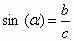If we want to determine what b equals to:

c . sin (a ) = b

Using a pocket calculator, we punch in the size of the angle 70, pressing the sine function to get 0,93969….. This gives us the value for sine a , which corresponds to the ratio of side b to side c. Now we multiply this by the length of side c.

0,93969 . 6 cm = 5,63815 cm

The result, which is the length of side b, we round up to 5.64 cm.

To find the size of angle a with the following information:

c = 8
b = 5

we use the following equation: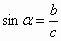The sine argument can be solved by using the opposite function, which is called the arc sine, to give use the following equation: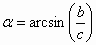First we calculate the fraction:From the value 0.625, we find out the arc sine, which is the angle which its sine is equal to this value. (On your calculator, there should be a key marked arcsin or sin-1. Otherwise, sometimes this function is activated by holding down the shift, INV or the 2nd key.)

For angle a , we get the value 38,68218….

The opposite functions for cosines and tangents are called arccosine and arctangent.

General Triangles

When calculating the length of sides and the size of angles of general triangles, more steps are necessary than with impedence triangles.

General triangles can be calculated using sine and cosine laws. The following sine law applies for the relation between angles and sides of triangles:

 In each triangle, the ratio of the length of each side to the opposing angle is always the same.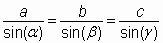When calculating this law, three quantities are necessary:

either two angles and one side which lies opposite one of the angles; or one angle, one side lying opposite it plus one other side. The sine law above can then be used to calculate the fourth value. Because only the length of the side lying between two known angles is given, the third angle can be calculated by considering that the sum of all angles must equal 180°, after which we can continue according to the sine law (although we need two sides and an angle lying opposite one of the two sides).

Various formulations of the sine law: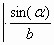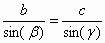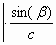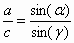a/sina = b/sinb / .sina /b a/b = sina /sinb

b/sinb = c/ ing / . sinb /c b/c = sinb /sing

a/sina = c/sing / . sina /c a/c = sina /sing

Cosine Law

If, in a triangle, we are given the length of two sides and the angle between them, we use the cosine law:

 The square of one side of a triangle equals the sum of the squares of the two other sides minus the double of the product of both those sides and the cosine of the angle opposite the first side.

c² = a² + b² – 2ab . cosg

According to which side we are trying to determine, the equation may look as any of the following:

b² = c² + a² – 2ac . cosb

a² = b² + c² – 2bc . cosa

If the angle is a right angle, its cosine equals zero. Then, from the cosine equation, a term of the cosine drops out to leave only Pythagora’s theorem.

Sine Table

the size of angle a 0° 10° 20° 30° 40° 50° 60° 70°

the length of the opposite leg to the hypotenuse 0 0,17 0,34 0,50 0,64 0,77 0,87 0,94

Cosine Table

the size of angle a 0° 10° 20° 30° 40° 50° 60° 70° 80°

the length of the adjacent leg to the hypotenuse 1 0,98 0,94 0,87 0,77 0,64 0,5 0,34 0,17

Tangent Table

The size of angle a 0° 10° 20° 30° 40° 50° 60° 70° 80°

The length of the adjacent leg to adjoining 0 0,18 0,36 0,58 0,84 1,19 1,73 2,74 5,67

Translating Dutch Hungarian Translations Hungarian Dutch Translating Swedish Czech Translations Czech Swedish Translating RussianEnter your search terms Submit search form